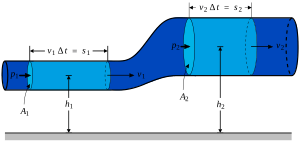# Newtonian fluid facts for kids

Kids Encyclopedia Facts
MechanicsA Newtonian fluid is a fluid, where the ratio between shear stress changes linearly in proportion to the stress it is exposed to. This proportion is known as viscosity. Note that the inverse is not true; there are fluids with constant viscosity, that are Non-Newtonian fluids: one example is the Boger fluid.Newtonian fluid Facts for Kids. Kiddle Encyclopedia.